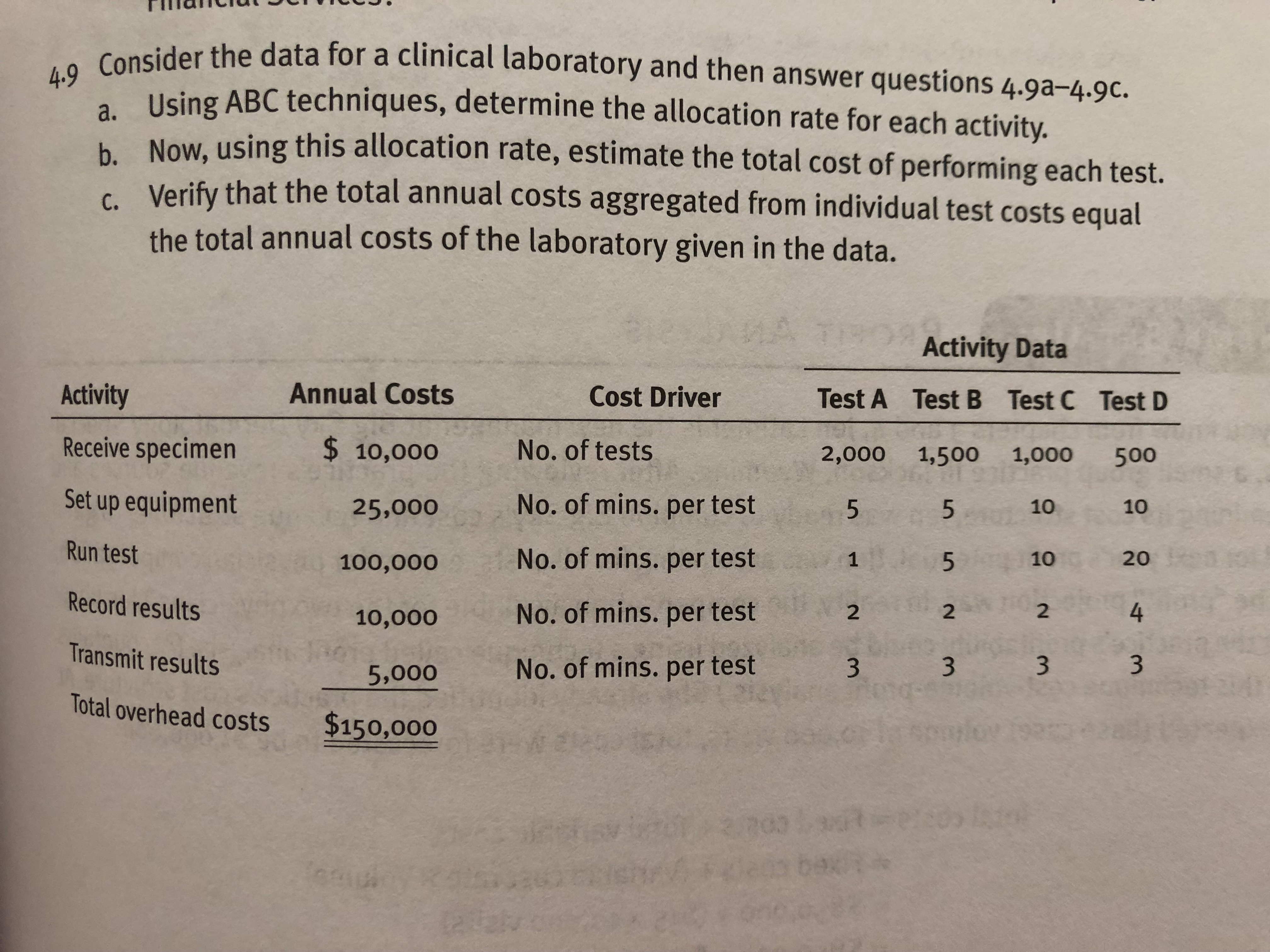# Consider the data for a clinical laboratory and then answer questions 4.9a-4.9c. Using ABC techniques, determine the allocation rate for each activity. Now, using this allocation rate, estimate the total cost of performing each test. Verify that the total annual costs aggregated from individual test costs equal the total annual costs of the laboratory given in the data. b. Activity Data Test A Test B Test C Test D 2,000 1,500 1,000 500 Cost Driver Annual Costs Activity Receive specimen Set up equipment Run test Record results No. of tests No. of mins. per test No. of mins. per test No. of mins. per test No. of mins. per test $10,000 25,000 100,000 10,000 Transmit results5,000 Total overhead costs$150,000 1010 20 4 3 10 1 2 2 2 3

Question

How to determine the allocation rate for each activity?help_outlineImage TranscriptioncloseConsider the data for a clinical laboratory and then answer questions 4.9a-4.9c. Using ABC techniques, determine the allocation rate for each activity. Now, using this allocation rate, estimate the total cost of performing each test. Verify that the total annual costs aggregated from individual test costs equal the total annual costs of the laboratory given in the data. b. Activity Data Test A Test B Test C Test D 2,000 1,500 1,000 500 Cost Driver Annual Costs Activity Receive specimen Set up equipment Run test Record results No. of tests No. of mins. per test No. of mins. per test No. of mins. per test No. of mins. per test $10,000 25,000 100,000 10,000 Transmit results5,000 Total overhead costs$150,000 1010 20 4 3 10 1 2 2 2 3 fullscreen